# Engineering Mechanics - Planar Kinematics of a Rigid Body (PKRB)

### Exercise :: Planar Kinematics of a Rigid Body (PKRB) - General Questions

• Planar Kinematics of a Rigid Body (PKRB) - General Questions
11.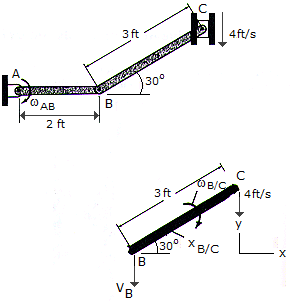If the block at C is moving downward at 4 ft/s, determine the angular velocity of bar AB at the instant shown.

 A. TAB = 2.00 rad/s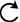B. TAB = 1.155 rad/sC. TAB = 0 D. TAB = 0.870 rad/sExplanation:

No answer description available for this question. Let us discuss.

12.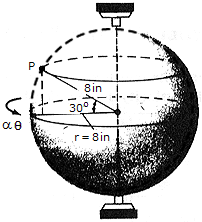The sphere starts from rest at= 0 and rotates with an angular acceleration of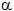= (4) rad/s2, whereis measured in radians. Determine the magnitudes of the velocity and acceleration of point P on the sphere at the instant= 6 rad.

 A. vP = 96.0 in./s, aP = 1168 in./s2 B. vP = 135.8 in./s, aP = 2310 in./s2 C. vP = 83.1 in./s, aP = 1011 in./s2 D. vP = 117.6 in./s, aP = 2000 in./s2

Explanation:

No answer description available for this question. Let us discuss.

13.Due to an engine failure, the missile is rotating at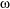= 3 rad/s, while its mass center G is moving upward at 200 ft/s. Determine the magnitude of the velocity of its nose B at this instant.

 A. vB = 214 ft/s B. vB = 125.0 ft/s C. vB = 275 ft/s D. vB = 185.4 ft/s

Explanation:

No answer description available for this question. Let us discuss.

14.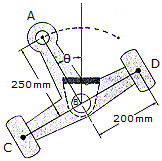Arm ABCD is printed at B and undergoes reciprocating motion such that= (0.3 sin 4t) rad, where t is measured in seconds and the argument for the sine is in radiaus. Determine the largest speed of point A during the motion and the magnitude of the acceleration of point D at this instant.

 A. vAmax = 0.0600 m/s, aD = 1.002 m/s2 B. vAmax = 0.300 m/s, aD = 0.960 m/s2 C. vAmax = 0.0600 m/s, aD = 0.916 m/s2 D. vAmax = 0.300 m/s, aD = 0.288 m/s2

Explanation:

No answer description available for this question. Let us discuss.

15.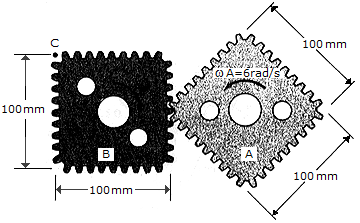At the instant shown, gear A is rotating with a constant angular velocity ofA = 6 rad/s. Determine the largest angular velocity of gear B and the maximum speed of point C.

 A.Bmax = 3.00 rad/s, vCmax = 0.212 m/s B.Bmax = 6.00 rad/s, vCmax = 0.424 m/s C.Bmax = 8.49 rad/s, vCmax = 0.600 m/s D.Bmax = 4.24 rad/s, vCmax = 0.300 m/s